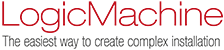Average 4 byte float - Printable Version +- Logic Machine Forum (https://forum.logicmachine.net) +-- Forum: LogicMachine eco-system (https://forum.logicmachine.net/forumdisplay.php?fid=1) +--- Forum: Scripting (https://forum.logicmachine.net/forumdisplay.php?fid=8) +--- Thread: Average 4 byte float (/showthread.php?tid=3292) Average 4 byte float - Tokatubs - 07.04.2021 Have used this with calculation with 2 byte float. But now i wanted it to calculate  L2, since my Kampstup meter only shows value on L1 and L2.  But not getting any values. Guess its could be because it is 4 byte float and not 2 byte. But what would be the correct value to use.  T1 = grp.getvalue('26/0/100') T2 = grp.getvalue('26/0/101') AV = math.round((T1 + T2) / 2, 0) grp.write('27/7/12', AV) I solved it in FBEditor using addition and division block but seems a bit "old fashion".RE: Average 4 byte float - admin - 08.04.2021 Try logging the values in your script: Code:```T1 = grp.getvalue('26/0/100') T2 = grp.getvalue('26/0/101') AV = math.round((T1 + T2) / 2, 0) log(T1, T2, AV) grp.write('27/7/12', AV)```